1548053061

# How to Remove Array Duplicates in ES6

Here are three ways to filter out duplicates from an array and return only the unique values. My favorite is using Set cause it’s the shortest and simplest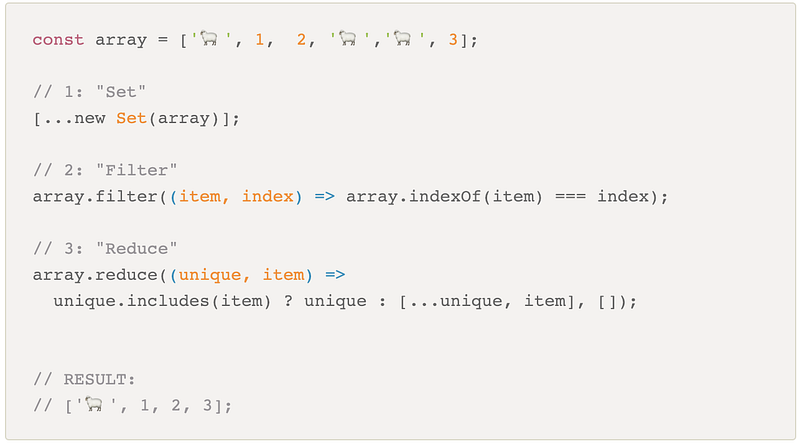### 1. Using Set

Let me start first by explaining what Set is:

Set is a new data object introduced in ES6. Because Set only lets you store unique values. When you pass in an array, it will remove any duplicate values.

Okay, let’s go back to our code and break down what’s happening. There are 2 things going on:

1. First, we are creating a new Setby passing an array. Because Setonly allows unique values, all duplicates will be removed.
2. Now the duplicates are gone, we’re going to convert it back to an array by using the spread operator ...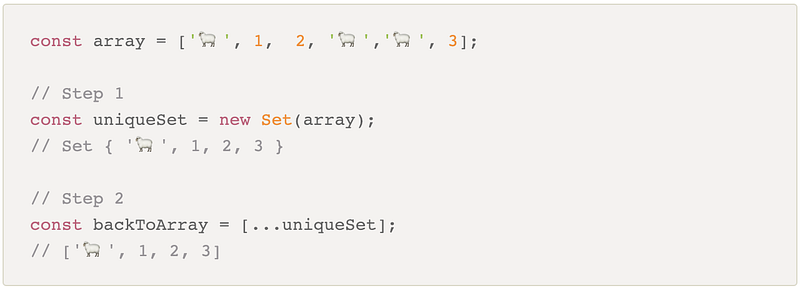#### Convert Set to an Array using Array.from

Alternatively, you can also use Array.from to convert a Set into an array: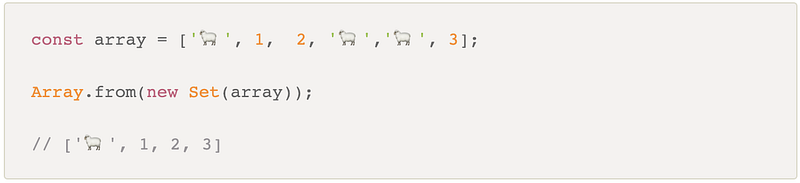### 2: Using filter

In order to understand this option, let’s go through what these two methods are doing: indexOf and filter.

#### indexOf

The indexOf method returns the first index it finds of the provided element from our array.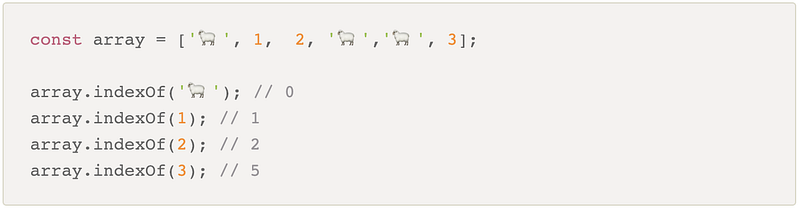#### filter

The filter() method creates a new array of elements that pass the conditional we provide. In other words, if the element passes and returns true, it will be included in the filtered array. And any element that fails or return false, it will be NOT be in the filtered array.

Let’s step in and walk through what happens as we loop through the array.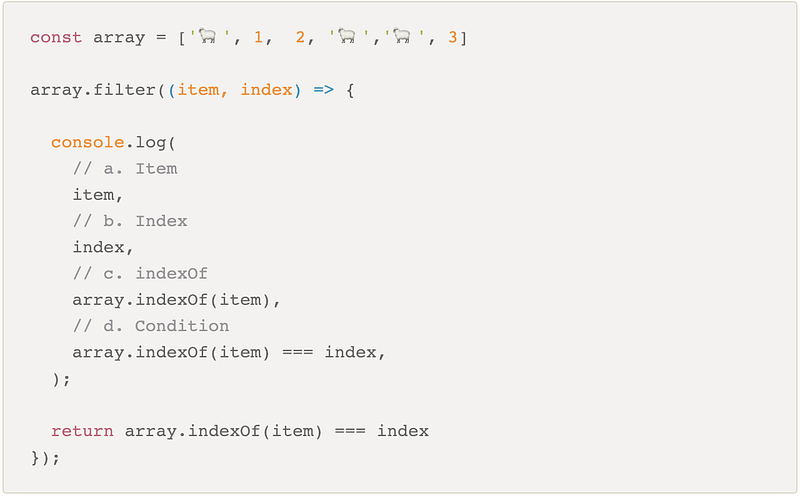Below is the output from the console.log showed above. The duplicates are where the index doesn’t match the indexOf. So in those cases, the condition will be false and won’t be included in our filtered array.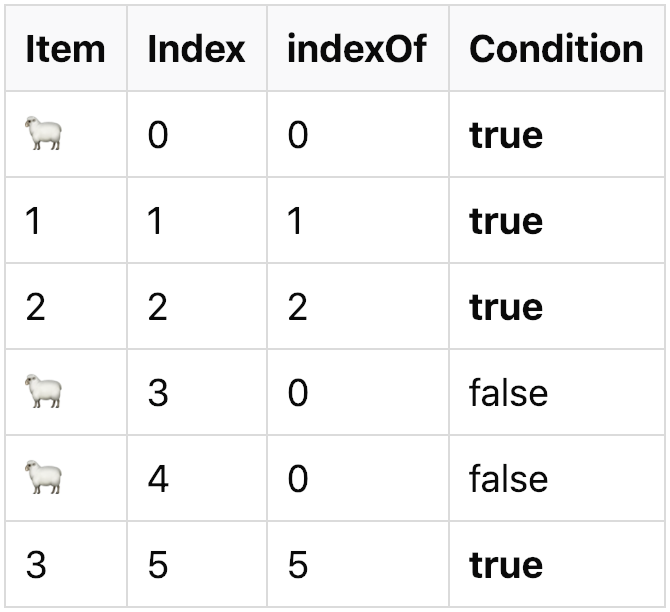#### Retrieve the duplicate values

We can also use the filter method to retrieve the duplicate values from the array. We can do this by simply adjusting our condition like so: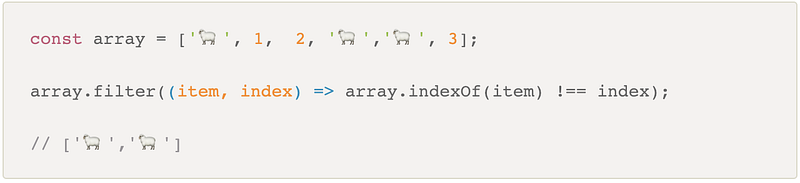Again, if we step through the code above and see the output: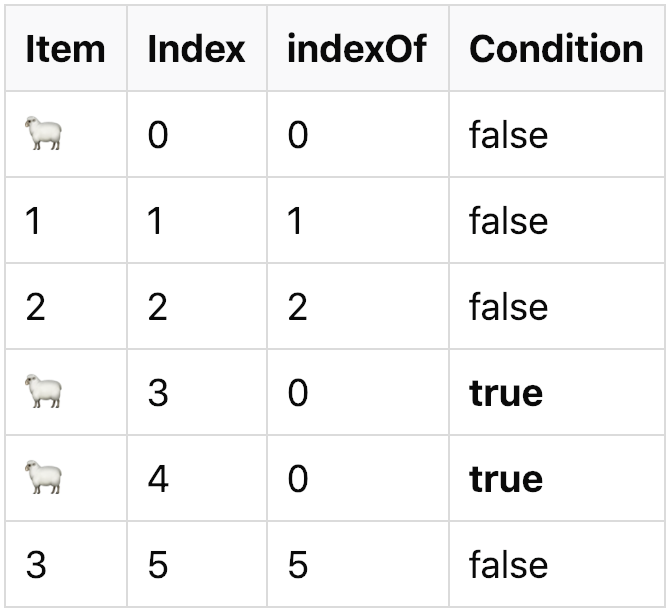### 3: Using reduce

The reduce method is used to reduce the elements of the array and combine them into a final array based on some reducer function that you pass.

In this case, our reducer function is checking if our final array contains the item. If it doesn’t, push that item into our final array. Otherwise, skip that element and return just our final array as is (essentially skipping over that element).

Reduce is always a bit more tricky to understand, so let’s also step into each case and see the output: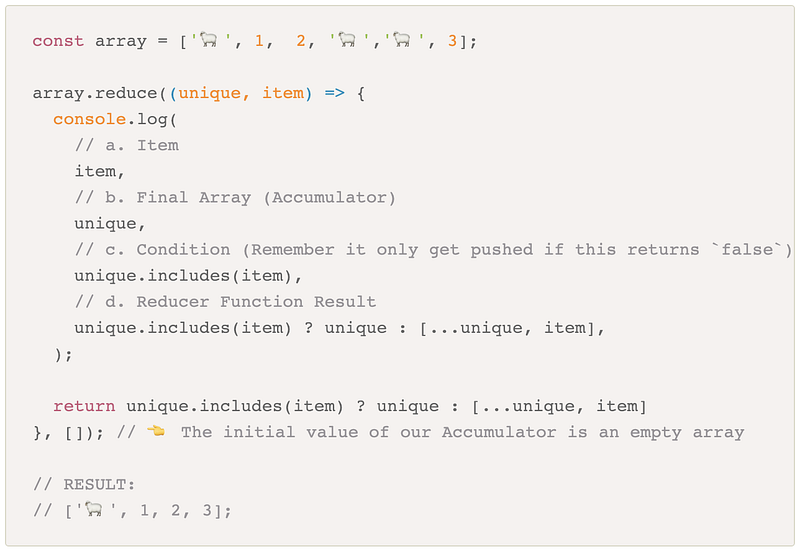And here’s the output from the console.log: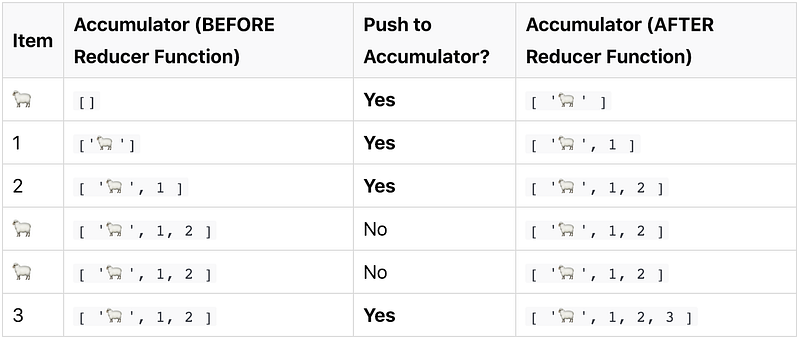### Community Input

By : Samantha Ming

#javascript

## Buddha Community1670560264

## Understanding Arrays in Python

### Learn how to use Python arrays. Create arrays in Python using the array module. You'll see how to define them and the different methods commonly used for performing operations on them.

The artcile covers arrays that you create by importing the `array module`. We won't cover NumPy arrays here.

1. Introduction to Arrays
1. The differences between Lists and Arrays
2. When to use arrays
2. How to use arrays
1. Define arrays
2. Find the length of arrays
3. Array indexing
4. Search through arrays
5. Loop through arrays
6. Slice an array
3. Array methods for performing operations
1. Change an existing value
3. Remove a value
4. Conclusion

Let's get started!

## What are Python Arrays?

Arrays are a fundamental data structure, and an important part of most programming languages. In Python, they are containers which are able to store more than one item at the same time.

Specifically, they are an ordered collection of elements with every value being of the same data type. That is the most important thing to remember about Python arrays - the fact that they can only hold a sequence of multiple items that are of the same type.

### What's the Difference between Python Lists and Python Arrays?

Lists are one of the most common data structures in Python, and a core part of the language.

Lists and arrays behave similarly.

Just like arrays, lists are an ordered sequence of elements.

They are also mutable and not fixed in size, which means they can grow and shrink throughout the life of the program. Items can be added and removed, making them very flexible to work with.

However, lists and arrays are not the same thing.

Lists store items that are of various data types. This means that a list can contain integers, floating point numbers, strings, or any other Python data type, at the same time. That is not the case with arrays.

As mentioned in the section above, arrays store only items that are of the same single data type. There are arrays that contain only integers, or only floating point numbers, or only any other Python data type you want to use.

### When to Use Python Arrays

Lists are built into the Python programming language, whereas arrays aren't. Arrays are not a built-in data structure, and therefore need to be imported via the `array module` in order to be used.

Arrays of the `array module` are a thin wrapper over C arrays, and are useful when you want to work with homogeneous data.

They are also more compact and take up less memory and space which makes them more size efficient compared to lists.

If you want to perform mathematical calculations, then you should use NumPy arrays by importing the NumPy package. Besides that, you should just use Python arrays when you really need to, as lists work in a similar way and are more flexible to work with.

## How to Use Arrays in Python

In order to create Python arrays, you'll first have to import the `array module` which contains all the necassary functions.

There are three ways you can import the `array module`:

1. By using `import array` at the top of the file. This includes the module `array`. You would then go on to create an array using `array.array()`.
``````import array

#how you would create an array
array.array()
``````
1. Instead of having to type `array.array()` all the time, you could use `import array as arr` at the top of the file, instead of `import array` alone. You would then create an array by typing `arr.array()`. The `arr` acts as an alias name, with the array constructor then immediately following it.
``````import array as arr

#how you would create an array
arr.array()
``````
1. Lastly, you could also use `from array import *`, with `*` importing all the functionalities available. You would then create an array by writing the `array()` constructor alone.
``````from array import *

#how you would create an array
array()
``````

### How to Define Arrays in Python

Once you've imported the `array module`, you can then go on to define a Python array.

The general syntax for creating an array looks like this:

``````variable_name = array(typecode,[elements])
``````

Let's break it down:

• `variable_name` would be the name of the array.
• The `typecode` specifies what kind of elements would be stored in the array. Whether it would be an array of integers, an array of floats or an array of any other Python data type. Remember that all elements should be of the same data type.
• Inside square brackets you mention the `elements` that would be stored in the array, with each element being separated by a comma. You can also create an empty array by just writing `variable_name = array(typecode)` alone, without any elements.

Below is a typecode table, with the different typecodes that can be used with the different data types when defining Python arrays:

Tying everything together, here is an example of how you would define an array in Python:

``````import array as arr

numbers = arr.array('i',[10,20,30])

print(numbers)

#output

#array('i', [10, 20, 30])
``````

Let's break it down:

• First we included the array module, in this case with `import array as arr `.
• Then, we created a `numbers` array.
• We used `arr.array()` because of `import array as arr `.
• Inside the `array()` constructor, we first included `i`, for signed integer. Signed integer means that the array can include positive and negative values. Unsigned integer, with `H` for example, would mean that no negative values are allowed.
• Lastly, we included the values to be stored in the array in square brackets.

Keep in mind that if you tried to include values that were not of `i` typecode, meaning they were not integer values, you would get an error:

``````import array as arr

numbers = arr.array('i',[10.0,20,30])

print(numbers)

#output

#Traceback (most recent call last):
# File "/Users/dionysialemonaki/python_articles/demo.py", line 14, in <module>
#   numbers = arr.array('i',[10.0,20,30])
#TypeError: 'float' object cannot be interpreted as an integer
``````

In the example above, I tried to include a floating point number in the array. I got an error because this is meant to be an integer array only.

Another way to create an array is the following:

``````from array import *

#an array of floating point values
numbers = array('d',[10.0,20.0,30.0])

print(numbers)

#output

#array('d', [10.0, 20.0, 30.0])
``````

The example above imported the `array module` via `from array import *` and created an array `numbers` of float data type. This means that it holds only floating point numbers, which is specified with the `'d'` typecode.

### How to Find the Length of an Array in Python

To find out the exact number of elements contained in an array, use the built-in `len()` method.

It will return the integer number that is equal to the total number of elements in the array you specify.

``````import array as arr

numbers = arr.array('i',[10,20,30])

print(len(numbers))

#output
# 3
``````

In the example above, the array contained three elements – `10, 20, 30` – so the length of `numbers` is `3`.

### Array Indexing and How to Access Individual Items in an Array in Python

Each item in an array has a specific address. Individual items are accessed by referencing their index number.

Indexing in Python, and in all programming languages and computing in general, starts at `0`. It is important to remember that counting starts at `0` and not at `1`.

To access an element, you first write the name of the array followed by square brackets. Inside the square brackets you include the item's index number.

The general syntax would look something like this:

``````array_name[index_value_of_item]
``````

Here is how you would access each individual element in an array:

``````import array as arr

numbers = arr.array('i',[10,20,30])

print(numbers) # gets the 1st element
print(numbers) # gets the 2nd element
print(numbers) # gets the 3rd element

#output

#10
#20
#30
``````

Remember that the index value of the last element of an array is always one less than the length of the array. Where `n` is the length of the array, `n - 1` will be the index value of the last item.

Note that you can also access each individual element using negative indexing.

With negative indexing, the last element would have an index of `-1`, the second to last element would have an index of `-2`, and so on.

Here is how you would get each item in an array using that method:

``````import array as arr

numbers = arr.array('i',[10,20,30])

print(numbers[-1]) #gets last item
print(numbers[-2]) #gets second to last item
print(numbers[-3]) #gets first item

#output

#30
#20
#10
``````

### How to Search Through an Array in Python

You can find out an element's index number by using the `index()` method.

You pass the value of the element being searched as the argument to the method, and the element's index number is returned.

``````import array as arr

numbers = arr.array('i',[10,20,30])

#search for the index of the value 10
print(numbers.index(10))

#output

#0
``````

If there is more than one element with the same value, the index of the first instance of the value will be returned:

``````import array as arr

numbers = arr.array('i',[10,20,30,10,20,30])

#search for the index of the value 10
#will return the index number of the first instance of the value 10
print(numbers.index(10))

#output

#0
``````

### How to Loop through an Array in Python

You've seen how to access each individual element in an array and print it out on its own.

You've also seen how to print the array, using the `print()` method. That method gives the following result:

``````import array as arr

numbers = arr.array('i',[10,20,30])

print(numbers)

#output

#array('i', [10, 20, 30])
``````

What if you want to print each value one by one?

This is where a loop comes in handy. You can loop through the array and print out each value, one-by-one, with each loop iteration.

For this you can use a simple `for` loop:

``````import array as arr

numbers = arr.array('i',[10,20,30])

for number in numbers:
print(number)

#output
#10
#20
#30
``````

You could also use the `range()` function, and pass the `len()` method as its parameter. This would give the same result as above:

``````import array as arr

values = arr.array('i',[10,20,30])

#prints each individual value in the array
for value in range(len(values)):
print(values[value])

#output

#10
#20
#30
``````

### How to Slice an Array in Python

To access a specific range of values inside the array, use the slicing operator, which is a colon `:`.

When using the slicing operator and you only include one value, the counting starts from `0` by default. It gets the first item, and goes up to but not including the index number you specify.

``````
import array as arr

#original array
numbers = arr.array('i',[10,20,30])

#get the values 10 and 20 only
print(numbers[:2])  #first to second position

#output

#array('i', [10, 20])
``````

When you pass two numbers as arguments, you specify a range of numbers. In this case, the counting starts at the position of the first number in the range, and up to but not including the second one:

``````import array as arr

#original array
numbers = arr.array('i',[10,20,30])

#get the values 20 and 30 only
print(numbers[1:3]) #second to third position

#output

#rray('i', [20, 30])
``````

## Methods For Performing Operations on Arrays in Python

Arrays are mutable, which means they are changeable. You can change the value of the different items, add new ones, or remove any you don't want in your program anymore.

Let's see some of the most commonly used methods which are used for performing operations on arrays.

### How to Change the Value of an Item in an Array

You can change the value of a specific element by speficying its position and assigning it a new value:

``````import array as arr

#original array
numbers = arr.array('i',[10,20,30])

#change the first element
#change it from having a value of 10 to having a value of 40
numbers = 40

print(numbers)

#output

#array('i', [40, 20, 30])
``````

### How to Add a New Value to an Array

To add one single value at the end of an array, use the `append()` method:

``````import array as arr

#original array
numbers = arr.array('i',[10,20,30])

#add the integer 40 to the end of numbers
numbers.append(40)

print(numbers)

#output

#array('i', [10, 20, 30, 40])
``````

Be aware that the new item you add needs to be the same data type as the rest of the items in the array.

Look what happens when I try to add a float to an array of integers:

``````import array as arr

#original array
numbers = arr.array('i',[10,20,30])

#add the integer 40 to the end of numbers
numbers.append(40.0)

print(numbers)

#output

#Traceback (most recent call last):
#  File "/Users/dionysialemonaki/python_articles/demo.py", line 19, in <module>
#   numbers.append(40.0)
#TypeError: 'float' object cannot be interpreted as an integer
``````

But what if you want to add more than one value to the end an array?

Use the `extend()` method, which takes an iterable (such as a list of items) as an argument. Again, make sure that the new items are all the same data type.

``````import array as arr

#original array
numbers = arr.array('i',[10,20,30])

#add the integers 40,50,60 to the end of numbers
#The numbers need to be enclosed in square brackets

numbers.extend([40,50,60])

print(numbers)

#output

#array('i', [10, 20, 30, 40, 50, 60])
``````

And what if you don't want to add an item to the end of an array? Use the `insert()` method, to add an item at a specific position.

The `insert()` function takes two arguments: the index number of the position the new element will be inserted, and the value of the new element.

``````import array as arr

#original array
numbers = arr.array('i',[10,20,30])

#add the integer 40 in the first position
#remember indexing starts at 0

numbers.insert(0,40)

print(numbers)

#output

#array('i', [40, 10, 20, 30])
``````

### How to Remove a Value from an Array

To remove an element from an array, use the `remove()` method and include the value as an argument to the method.

``````import array as arr

#original array
numbers = arr.array('i',[10,20,30])

numbers.remove(10)

print(numbers)

#output

#array('i', [20, 30])
``````

With `remove()`, only the first instance of the value you pass as an argument will be removed.

See what happens when there are more than one identical values:

``````
import array as arr

#original array
numbers = arr.array('i',[10,20,30,10,20])

numbers.remove(10)

print(numbers)

#output

#array('i', [20, 30, 10, 20])
``````

Only the first occurence of `10` is removed.

You can also use the `pop()` method, and specify the position of the element to be removed:

``````import array as arr

#original array
numbers = arr.array('i',[10,20,30,10,20])

#remove the first instance of 10
numbers.pop(0)

print(numbers)

#output

#array('i', [20, 30, 10, 20])
``````

## Conclusion

And there you have it - you now know the basics of how to create arrays in Python using the `array module`. Hopefully you found this guide helpful.

You'll start from the basics and learn in an interacitve and beginner-friendly way. You'll also build five projects at the end to put into practice and help reinforce what you learned.

Thanks for reading and happy coding!

Original article source at https://www.freecodecamp.org

#python1666082925

## How to Create Arrays in Python

### In this tutorial, you'll know the basics of how to create arrays in Python using the array module. Learn how to use Python arrays. You'll see how to define them and the different methods commonly used for performing operations on them.

This tutorialvideo on 'Arrays in Python' will help you establish a strong hold on all the fundamentals in python programming language. Below are the topics covered in this video:
1:15 What is an array?
2:53 Is python list same as an array?
3:48  How to create arrays in python?
7:19 Accessing array elements
9:59 Basic array operations
- 10:33  Finding the length of an array
- 15:06  Removing elements
- 18:32  Array concatenation
- 20:59  Slicing
- 23:26  Looping

Python Array Tutorial – Define, Index, Methods

In this article, you'll learn how to use Python arrays. You'll see how to define them and the different methods commonly used for performing operations on them.

The artcile covers arrays that you create by importing the `array module`. We won't cover NumPy arrays here.

1. Introduction to Arrays
1. The differences between Lists and Arrays
2. When to use arrays
2. How to use arrays
1. Define arrays
2. Find the length of arrays
3. Array indexing
4. Search through arrays
5. Loop through arrays
6. Slice an array
3. Array methods for performing operations
1. Change an existing value
3. Remove a value
4. Conclusion

Let's get started!

## What are Python Arrays?

Arrays are a fundamental data structure, and an important part of most programming languages. In Python, they are containers which are able to store more than one item at the same time.

Specifically, they are an ordered collection of elements with every value being of the same data type. That is the most important thing to remember about Python arrays - the fact that they can only hold a sequence of multiple items that are of the same type.

### What's the Difference between Python Lists and Python Arrays?

Lists are one of the most common data structures in Python, and a core part of the language.

Lists and arrays behave similarly.

Just like arrays, lists are an ordered sequence of elements.

They are also mutable and not fixed in size, which means they can grow and shrink throughout the life of the program. Items can be added and removed, making them very flexible to work with.

However, lists and arrays are not the same thing.

Lists store items that are of various data types. This means that a list can contain integers, floating point numbers, strings, or any other Python data type, at the same time. That is not the case with arrays.

As mentioned in the section above, arrays store only items that are of the same single data type. There are arrays that contain only integers, or only floating point numbers, or only any other Python data type you want to use.

### When to Use Python Arrays

Lists are built into the Python programming language, whereas arrays aren't. Arrays are not a built-in data structure, and therefore need to be imported via the `array module` in order to be used.

Arrays of the `array module` are a thin wrapper over C arrays, and are useful when you want to work with homogeneous data.

They are also more compact and take up less memory and space which makes them more size efficient compared to lists.

If you want to perform mathematical calculations, then you should use NumPy arrays by importing the NumPy package. Besides that, you should just use Python arrays when you really need to, as lists work in a similar way and are more flexible to work with.

## How to Use Arrays in Python

In order to create Python arrays, you'll first have to import the `array module` which contains all the necassary functions.

There are three ways you can import the `array module`:

• By using `import array` at the top of the file. This includes the module `array`. You would then go on to create an array using `array.array()`.
``````import array

#how you would create an array
array.array()``````
• Instead of having to type `array.array()` all the time, you could use `import array as arr` at the top of the file, instead of `import array` alone. You would then create an array by typing `arr.array()`. The `arr` acts as an alias name, with the array constructor then immediately following it.
``````import array as arr

#how you would create an array
arr.array()``````
• Lastly, you could also use `from array import *`, with `*` importing all the functionalities available. You would then create an array by writing the `array()` constructor alone.
``````from array import *

#how you would create an array
array()``````

### How to Define Arrays in Python

Once you've imported the `array module`, you can then go on to define a Python array.

The general syntax for creating an array looks like this:

``variable_name = array(typecode,[elements])``

Let's break it down:

• `variable_name` would be the name of the array.
• The `typecode` specifies what kind of elements would be stored in the array. Whether it would be an array of integers, an array of floats or an array of any other Python data type. Remember that all elements should be of the same data type.
• Inside square brackets you mention the `elements` that would be stored in the array, with each element being separated by a comma. You can also create an empty array by just writing `variable_name = array(typecode)` alone, without any elements.

Below is a typecode table, with the different typecodes that can be used with the different data types when defining Python arrays:

Tying everything together, here is an example of how you would define an array in Python:

``````import array as arr

numbers = arr.array('i',[10,20,30])

print(numbers)

#output

#array('i', [10, 20, 30])``````

Let's break it down:

• First we included the array module, in this case with `import array as arr `.
• Then, we created a `numbers` array.
• We used `arr.array()` because of `import array as arr `.
• Inside the `array()` constructor, we first included `i`, for signed integer. Signed integer means that the array can include positive and negative values. Unsigned integer, with `H` for example, would mean that no negative values are allowed.
• Lastly, we included the values to be stored in the array in square brackets.

Keep in mind that if you tried to include values that were not of `i` typecode, meaning they were not integer values, you would get an error:

``````import array as arr

numbers = arr.array('i',[10.0,20,30])

print(numbers)

#output

#Traceback (most recent call last):
# File "/Users/dionysialemonaki/python_articles/demo.py", line 14, in <module>
#   numbers = arr.array('i',[10.0,20,30])
#TypeError: 'float' object cannot be interpreted as an integer``````

In the example above, I tried to include a floating point number in the array. I got an error because this is meant to be an integer array only.

Another way to create an array is the following:

``````from array import *

#an array of floating point values
numbers = array('d',[10.0,20.0,30.0])

print(numbers)

#output

#array('d', [10.0, 20.0, 30.0])``````

The example above imported the `array module` via `from array import *` and created an array `numbers` of float data type. This means that it holds only floating point numbers, which is specified with the `'d'` typecode.

### How to Find the Length of an Array in Python

To find out the exact number of elements contained in an array, use the built-in `len()` method.

It will return the integer number that is equal to the total number of elements in the array you specify.

``````import array as arr

numbers = arr.array('i',[10,20,30])

print(len(numbers))

#output
# 3``````

In the example above, the array contained three elements – `10, 20, 30` – so the length of `numbers` is `3`.

### Array Indexing and How to Access Individual Items in an Array in Python

Each item in an array has a specific address. Individual items are accessed by referencing their index number.

Indexing in Python, and in all programming languages and computing in general, starts at `0`. It is important to remember that counting starts at `0` and not at `1`.

To access an element, you first write the name of the array followed by square brackets. Inside the square brackets you include the item's index number.

The general syntax would look something like this:

``array_name[index_value_of_item]``

Here is how you would access each individual element in an array:

``````import array as arr

numbers = arr.array('i',[10,20,30])

print(numbers) # gets the 1st element
print(numbers) # gets the 2nd element
print(numbers) # gets the 3rd element

#output

#10
#20
#30``````

Remember that the index value of the last element of an array is always one less than the length of the array. Where `n` is the length of the array, `n - 1` will be the index value of the last item.

Note that you can also access each individual element using negative indexing.

With negative indexing, the last element would have an index of `-1`, the second to last element would have an index of `-2`, and so on.

Here is how you would get each item in an array using that method:

``````import array as arr

numbers = arr.array('i',[10,20,30])

print(numbers[-1]) #gets last item
print(numbers[-2]) #gets second to last item
print(numbers[-3]) #gets first item

#output

#30
#20
#10``````

### How to Search Through an Array in Python

You can find out an element's index number by using the `index()` method.

You pass the value of the element being searched as the argument to the method, and the element's index number is returned.

``````import array as arr

numbers = arr.array('i',[10,20,30])

#search for the index of the value 10
print(numbers.index(10))

#output

#0``````

If there is more than one element with the same value, the index of the first instance of the value will be returned:

``````import array as arr

numbers = arr.array('i',[10,20,30,10,20,30])

#search for the index of the value 10
#will return the index number of the first instance of the value 10
print(numbers.index(10))

#output

#0``````

### How to Loop through an Array in Python

You've seen how to access each individual element in an array and print it out on its own.

You've also seen how to print the array, using the `print()` method. That method gives the following result:

``````import array as arr

numbers = arr.array('i',[10,20,30])

print(numbers)

#output

#array('i', [10, 20, 30])``````

What if you want to print each value one by one?

This is where a loop comes in handy. You can loop through the array and print out each value, one-by-one, with each loop iteration.

For this you can use a simple `for` loop:

``````import array as arr

numbers = arr.array('i',[10,20,30])

for number in numbers:
print(number)

#output
#10
#20
#30``````

You could also use the `range()` function, and pass the `len()` method as its parameter. This would give the same result as above:

``````import array as arr

values = arr.array('i',[10,20,30])

#prints each individual value in the array
for value in range(len(values)):
print(values[value])

#output

#10
#20
#30``````

### How to Slice an Array in Python

To access a specific range of values inside the array, use the slicing operator, which is a colon `:`.

When using the slicing operator and you only include one value, the counting starts from `0` by default. It gets the first item, and goes up to but not including the index number you specify.

``````import array as arr

#original array
numbers = arr.array('i',[10,20,30])

#get the values 10 and 20 only
print(numbers[:2])  #first to second position

#output

#array('i', [10, 20])``````

When you pass two numbers as arguments, you specify a range of numbers. In this case, the counting starts at the position of the first number in the range, and up to but not including the second one:

``````import array as arr

#original array
numbers = arr.array('i',[10,20,30])

#get the values 20 and 30 only
print(numbers[1:3]) #second to third position

#output

#rray('i', [20, 30])``````

## Methods For Performing Operations on Arrays in Python

Arrays are mutable, which means they are changeable. You can change the value of the different items, add new ones, or remove any you don't want in your program anymore.

Let's see some of the most commonly used methods which are used for performing operations on arrays.

### How to Change the Value of an Item in an Array

You can change the value of a specific element by speficying its position and assigning it a new value:

``````import array as arr

#original array
numbers = arr.array('i',[10,20,30])

#change the first element
#change it from having a value of 10 to having a value of 40
numbers = 40

print(numbers)

#output

#array('i', [40, 20, 30])``````

### How to Add a New Value to an Array

To add one single value at the end of an array, use the `append()` method:

``````import array as arr

#original array
numbers = arr.array('i',[10,20,30])

#add the integer 40 to the end of numbers
numbers.append(40)

print(numbers)

#output

#array('i', [10, 20, 30, 40])``````

Be aware that the new item you add needs to be the same data type as the rest of the items in the array.

Look what happens when I try to add a float to an array of integers:

``````import array as arr

#original array
numbers = arr.array('i',[10,20,30])

#add the integer 40 to the end of numbers
numbers.append(40.0)

print(numbers)

#output

#Traceback (most recent call last):
#  File "/Users/dionysialemonaki/python_articles/demo.py", line 19, in <module>
#   numbers.append(40.0)
#TypeError: 'float' object cannot be interpreted as an integer``````

But what if you want to add more than one value to the end an array?

Use the `extend()` method, which takes an iterable (such as a list of items) as an argument. Again, make sure that the new items are all the same data type.

``````import array as arr

#original array
numbers = arr.array('i',[10,20,30])

#add the integers 40,50,60 to the end of numbers
#The numbers need to be enclosed in square brackets

numbers.extend([40,50,60])

print(numbers)

#output

#array('i', [10, 20, 30, 40, 50, 60])``````

And what if you don't want to add an item to the end of an array? Use the `insert()` method, to add an item at a specific position.

The `insert()` function takes two arguments: the index number of the position the new element will be inserted, and the value of the new element.

``````import array as arr

#original array
numbers = arr.array('i',[10,20,30])

#add the integer 40 in the first position
#remember indexing starts at 0

numbers.insert(0,40)

print(numbers)

#output

#array('i', [40, 10, 20, 30])``````

### How to Remove a Value from an Array

To remove an element from an array, use the `remove()` method and include the value as an argument to the method.

``````import array as arr

#original array
numbers = arr.array('i',[10,20,30])

numbers.remove(10)

print(numbers)

#output

#array('i', [20, 30])``````

With `remove()`, only the first instance of the value you pass as an argument will be removed.

See what happens when there are more than one identical values:

``````import array as arr

#original array
numbers = arr.array('i',[10,20,30,10,20])

numbers.remove(10)

print(numbers)

#output

#array('i', [20, 30, 10, 20])``````

Only the first occurence of `10` is removed.

You can also use the `pop()` method, and specify the position of the element to be removed:

``````import array as arr

#original array
numbers = arr.array('i',[10,20,30,10,20])

#remove the first instance of 10
numbers.pop(0)

print(numbers)

#output

#array('i', [20, 30, 10, 20])``````

## Conclusion

And there you have it - you now know the basics of how to create arrays in Python using the `array module`. Hopefully you found this guide helpful.

Thanks for reading and happy coding!

#python #programming1623911281

## How to Print an Array in Java

#### Introduction

Printing an array is a quick way to give us visibility on the values of the contents inside. Sometimes the array values are the desired output of the program.

In this article, we’ll take a look at how to print an array in Java using four different ways.

While the “best way” depends on what your program needs to do, we begin with the simplest method for printing and then show more verbose ways to do it.

#java #array #how to print an array in java #array in java #print an array in java #print1680014100

## How to Remove punctuation using Python

If you have ever worked processing a large amount of textual data, you would know the pain of finding and removing irrelevant words or characters from the text.l
Doing this job manually, even with the help of modern word processors, can be time-consuming and frustrating.
Fortunately, programming languages such as Python support powerful text processing libraries that help us do such clean-up jobs efficiently.
In this tutorial, we will look at various ways of removing punctuation from a text in Python.

## Why Remove punctuation?

Removing punctuation is a common preprocessing step in many data analysis and machine learning tasks.
For example, if you’re building a text classification model, or constructing a word cloud from a given text corpus, punctuation are of no use in such tasks and so we remove them at the pre-processing step.
If you’re working on user-generated text data such as social media posts, you’d encounter too much punctuation in the sentences, which may not be useful for the task at hand, and so removing all of them becomes an essential pre-processing task.

## Using replace method

Python strings come with many useful methods. One such method is the replace method.
Using this method, you can replace a specific character or substring in a given string with another character or substring.
Let us look at an example.

``````s = "Hello World, Welcome to my blog."
print(s)
s1 = s.replace('W', 'V')
print(s1)``````

Output:

This method, by default, removes all occurrences of a given character or substring from the given string.
We can limit the number of occurrences to replace by passing a ‘count’ value as the 3rd parameter to the replace method.

Here’s an example where we first use the default value of count(-1) and then pass a custom value for it.

``````s = "Hello world, Welcome to my blog."
print(s)
s1 = s.replace('o', 'a')
print(f"After replacing all o's with a's: {s1}")
# replace only first 2 o's
s2 = s.replace('o', 'a', 2)
print(f"After replacing first two o's: {s2}")
``````

Output:

It is important to note that in all our usages of the replace method, we’ve stored the result string in a new variable.
This is because strings are immutable. Unlike lists, we cannot modify them in place.
Hence, all string modification methods return a new, modified string that we store in a new variable.

Now let’s figure out how we should use this method to replace all occurrences of punctuation in a string.

We must first define a list of all punctuation that we are not interested in and want to get rid of.
We then iterate over each of these punctuations and pass it to the replace method called on the input string.
Also, since we want to remove the punctuation, we pass an empty string as the 2nd parameter to replace it.

``````user_comment = "NGL, i just loved the moviee...... excellent work !!!"
print(f"input string: {user_comment}")
clean_comment = user_comment #copy the string in new variable, we'll store the result in this variable
# define list of punctuation to be removed
punctuation = ['.','.','!']
# iteratively remove all occurrences of each punctuation in the input
for p in punctuation:
clean_comment = clean_comment.replace(p,'') #not specifying 3rd param, since we want to remove all occurrences
print(f"clean string: {clean_comment}")
``````

Output:

Since it was a short text, we could anticipate what kind of punctuation we would encounter.
But real-world inputs could span thousands of lines of texts, and it would be difficult to figure out which punctuation is present and need to be eliminated.
However, if we are aware of all the punctuation we may encounter in an English text, our task would become easy.
Python’s string class does provide all punctuation in the attribute string.punctuation. It’s a string of punctuation.

``````import string
all_punctuation = string.punctuation
print(f"All punctuation: {all_punctuation}")
``````

Output:

Once we have all the punctuation as a sequence of characters, we can run the previous for loop on any text input, however large, and the output will be free of punctuation.

## Using maketrans and translate

There is another way in Python using which we can replace all occurrences of a bunch of characters in a string by their corresponding equivalents as desired.
In this method, we first create a ‘translation table’ using str.translate. This table specifies a one-to-one mapping between characters.
We then pass this translation table to the translate method called on the input string.
This method returns a modified string where original characters are replaced by their replacements as defined in the translation table.

Let’s understand this through a simple example. We will replace all occurrences of ‘a’ with ‘e’, ‘o’ with ‘u’, and ‘i’ with ‘y’.

``````tr_table = str.maketrans('aoi', 'euy') #defining the translation table: a=>e, o=>u, i=>y
s = "i absolutely love the american ice-cream!"
print(f"Original string: {s}")
s1 = s.translate(tr_table) #or str.translate(s, tr_table)
print(f"Translated string: {s1}")
``````

Output:

In the maketrans method, the first two strings need to be of equal length, as each character in the 1st string corresponds to its replacement/translation in the 2nd string.
The method accepts an optional 3rd string parameter specifying characters that need to be mapped to None, meaning they don’t have replacements and hence will be removed (this is the functionality we need to remove punctuation).

We can also create the translation table using a dictionary of mappings instead of the two string parameters.

This additionally allows us to create character-to-strings mappings, which help us replace a single character with strings (which is impossible with string parameters).
The dictionary approach also helps us explicitly map any character(s) to None, indicating those characters need to be removed.

Let us use the previous example and create the mapping using a dictionary.
Now, we will also map ‘!’ to None, which will result in the removal of the punctuation from the input string.

``````mappings = {
'a':'e',
'o':'u',
'i':'eye',
'!': None
}
tr_table = str.maketrans(mappings)
s = "i absolutely love the american ice-cream!"
print(f"Original string: {s}")
print(f"translation table: {tr_table}")
s1 = s.translate(tr_table) #or str.translate(s, tr_table)
print(f"Translated string: {s1}")
``````

Output:

Note that when we print the translation table, the keys are integers instead of characters. These are the Unicode values of the characters we had defined when creating the table.

Finally, let’s use this approach to remove all punctuation occurrences from a given input text.

``````import string
s = """I reached at the front of the billing queue. The cashier started scanning my items, one after the other.
Off went from my cart the almonds, the butter, the sugar, the coffee.... when suddenly I heard an old lady, the 3rd in queue behind me, scream at me, "What y'all taking all day for ! are you hoarding for the whole year !".
The cashier looked tensed, she dashed all the remaining products as fast as she could, and then squeaked in a nervous tone, "That would be 298.5, sir !"."""
print(f"input string:\n{s}\n")
tr_table = str.maketrans("","", string.punctuation)
s1 = s.translate(tr_table)
print(f"translated string:\n{s1}\n")
``````

Output:

## Using RegEx

RegEx, or Regular Expression, is a sequence of characters representing a string pattern.
In text-processing, it is used to find, replace, or delete all such substrings that match the pattern defined by the regular expression.
For eg. the regex “\d{10}” is used to represent 10-digit numbers, or the regex “[A-Z]{3}” is used to represent any 3-letter(uppercase) code. Let us use this to find country codes from a sentence.

``````import re
# define regex pattern for 3-lettered country codes.
c_pattern = re.compile("[A-Z]{3}")
s = "At the Olympics, the code for Japan is JPN, and that of Brazil is BRA. RSA stands for the 'Republic of South Africa' while ARG for Argentina."
print(f"Input: {s}")
# find all substrings matching the above regex
countries = re.findall(c_pattern, s)
print(f"Countries fetched: {countries}")
``````

Output:

All occurrences of 3-lettered uppercase codes have been identified with the help of the regex we defined.

If we want to replace all the matching patterns in the string with something, we can do so using the re.sub method.
Let us try replacing all occurrences of the country codes with a default code “DEF” in the earlier example.

``````c_pattern = re.compile("[A-Z]{3}")
s = "At the Olympics, the code for Japan is JPN, and that of Brazil is BRA. RSA stands for the 'Republic of South Africa' while ARG for Argentina.\n"
print(f"Input:\n{s}")
new_s = re.sub(c_pattern, "DEF", s)
print(f"After replacement:\n{new_s}")
``````

Output:

We can use the same method to replace all occurrences of the punctuation with an empty string. This would effectively remove all the punctuation from the input string.
But first, we need to define a regex pattern that would represent all the punctuation.
While there doesn’t exist any special character for punctuation, like \d for digits, we can either explicitly define all the punctuation that we’d like to replace,
Or we can define a regex to exclude all the characters that we would like to retain.

For example, if we know that we can expect only the English alphabet, digits, and whitespace, then we can exclude them all in our regex using the caret symbol ^.
Everything else by default will be matched and replaced.

Let’s define it both ways.

``````import string, re
p_punct1 = re.compile(f"[{string.punctuation}]") #trivial way of regex for punctuation
print(f"regex 1 for punctuation: {p_punct1}")
p_punct2 = re.compile("[^\w\s]") #definition by exclusion
print(f"regex 2 for punctuation: {p_punct2}")
``````

Output:

Now let us use both of them to replace all the punctuation from a sentence. We’ll use an earlier sentence that contains various punctuation.

``````import string
s = """I reached at the front of the billing queue. The cashier started scanning my items, one after the other.
Off went from my cart the almonds, the butter, the sugar, the coffee.... when suddenly I heard an old lady, the 3rd in queue behind me, scream at me, "What y'all taking all day for ! are you hoarding for the whole year !".
The cashier looked tensed, she dashed all the remaining products as fast as she could, and then squeaked in a nervous tone, "That would be 298.5, sir !"."""
print(f"input string:\n{s}\n")
s1 = re.sub(p_punct1, "", s)
print(f"after removing punctuation using 1st regex:\n{s1}\n")
s2 = re.sub(p_punct2, "", s)
print(f"after removing punctuation using 2nd regex:\n{s2}\n")
``````

Output:

Both of them produced results identical to each other and to the maketrans method we used earlier.

## Using nltk

Python’s nltk is a popular, open-source NLP library. It offers a large range of language datasets, text-processing modules, and a host of other features required in NLP.
nltk has a method called word_tokenize, which is used to break the input sentence into a list of words. This is one of the first steps in any NLP pipeline.
Let’s look at an example.

``````import nltk
s = "We can't lose this game so easily, not without putting up a fight!"
tokens = nltk.word_tokenize(s)
print(f"input: {s}")
print(f"tokens: {tokens}")
``````

Output:

The default tokenizer being used by nltk retains punctuation and splits the tokens based on whitespace and punctuation.

We can use nltk’s RegexpTokenizer to specify token patterns using regex.

``````from nltk.tokenize import RegexpTokenizer
tokenizer = RegexpTokenizer("\w+") #\w+ matches alphanumeric characters a-z,A-Z,0-9 and _
s = "We can't lose this game so easily, not without putting up a fight!"
tokens = tokenizer.tokenize(s)
print(f"input: {s}\n")
print(f"tokens: {tokens}\n")
new_s = " ".join(tokens)
print(f"New string: {new_s}\n")
``````

Output:

## Remove punctuation from start and end only

If we want to remove the punctuation only from the start and end of the sentence, and not those between, we can define a regex representing such a pattern and use it to remove the leading and the trailing punctuation.

Let’s first use one such regular expression in an example, and then we will dive deeper into that regex.

``````import re
pattern = re.compile("(^[^\w\s]+)|([^\w\s]+\$)")
sentence = '"I am going to be the best player in history!"'
print(sentence)
print(re.sub(pattern,"", sentence))
``````

Output:

The output shows the quotes (“) at the beginning and end, as well as the exclamation mark (!) at the second-to-last position, have been removed.
The punctuation occurring between the words, on the other hand, is retained.

The regex being used to achieve this is (^[^\w\s]+)|([^\w\s]+\$)

There are two, different patterns in this regex, each enclosed in parentheses and separated by an OR sign (|). That means, if either of the two patterns exists in the string, it will be identified by the given regex.
The first part of the regex is “^[^\w\s]+”. There are two caret signs (^) here, one inside the square brackets, and the other, outside.
The first caret i.e the one preceding the opening square bracket, tells the regex compiler to “match any substring that occurs at the BEGINNING of the sentence and matches the following pattern”.
The square brackets define a set of characters to match.
The caret inside the square bracket tells the compiler to “match everything EXCEPT \w and \s”. \w represents alphanumeric characters, and \s, whitespace.
Thus, everything at the beginning, other than alphanumeric characters and whitespace (which would essentially be the punctuation) will be represented by the first part of the regex.

The second component is almost similar to the first one, except that it matches the specified set of characters occurring AT THE END of the string. This is denoted by the trailing character \$.

## Remove punctuation and extra spaces

In addition to removing punctuation, removing extra spaces is a common preprocessing step.
Removing extra spaces doesn’t require the use of any regex or nltk method. Python string’s strip method is used to remove any leading or trailing whitespace characters.

``````s = " I have an idea! \t "
print(f"input string with white spaces = {s}, length = {len(s)}\n")
s1 = s.strip()
print(f"after removing spaces from both ends: {s1}, length = {len(s1)}")``````

Output:

The strip method removes white spaces only at the beginning and end of the string.
We would also like to remove the extra spaces between the words.
Both of these can be achieved by splitting the string with the split method, and then joining them using a single space ” “.

Let us combine the removal of punctuation and extra spaces in an example.

``````import string
tr_table = str.maketrans("","", string.punctuation) # for removing punctuation
s = '   "   I am going to be     the best,\t  the most-loved, and...    the richest player in history!  " '
print(f"Original string:\n{s},length = {len(s)}\n")
s = s.translate(tr_table)
print(f"After removing punctuation:\n{s},length = {len(s)}\n")
s = " ".join(s.split())
print(f"After removing extra spaces:\n{s},length = {len(s)}")
``````

Output:

## Remove punctuation from a text file

So far, we have been working on short strings that were stored in variables of type str and were no longer than 2-3 sentences.
But in the real world, the actual data may be stored in large files on the disk.
In this section, we will look at how to remove punctuation from a text file.

First, let’s read the whole content of the file in a string variable and use one of our earlier methods to remove the punctuation from this content string before writing it into a new file.

``````import re
punct = re.compile("[^\w\s]")
input_file = "short_sample.txt"
output_file = "short_sample_processed.txt"
f = open(input_file)
print(f"File content: {file_content}\n")
new_file_content = re.sub(punct, "", file_content)
print(f"New file content: {new_file_content}\n")
# writing it to new file
with open(output_file, "w") as fw:
fw.write(new_file_content)
``````

Output:

We read the entire file at once in the above example. The text file, however, may also span content up to millions of lines, amounting to a few hundred MBs or a few GBs.
In such a case, it doesn’t make sense to read the entire file at once, as that could lead to potential memory overload errors.

So, we will read the text file one line at a time, process it, and write it to the new file.
Doing this iteratively will not cause memory overload, however, it may add some overhead because repetitive input/output operations are costlier.

In the following example, we will remove punctuation from a text file(found here), which is a story about ‘The Devil With Three Golden Hairs’!

``````import re
punct = re.compile("[^\w\s]")
input_file = "the devil with three golden hairs.txt"
output_file = "the devil with three golden hairs_processed.txt"
# writing it to new file
with open(output_file, "w") as f_writer:
line = line.strip() #removing whitespace at ends
line = re.sub(punct, "",line) #removing punctuation
line += "\n"
f_writer.write(line)
print(f"First 10 lines of original file:")
with open(input_file) as f:
i = 0
for line in f:
print(line,end="")
i+=1
if i==10:
break
print(f"\nFirst 10 lines of output file:")
with open(output_file) as f:
i = 0
for line in f:
print(line,end="")
i+=1
if i==10:
break
``````

Output:

As seen from the first 10 lines, the punctuation has been removed from the input file, and the result is stored in the output file.

## Remove all punctuation except apostrophe

Apostrophes, in the English language, carry semantic meanings. They are used to show possessive nouns, to shorten words by the omission of letters (eg. cannot=can’t, will not=won’t), etc.

So it becomes important to retain the apostrophe characters while processing texts to avoid losing these semantic meanings.

Let us remove all the punctuation but the apostrophes from a text.

``````s=""""I should like to have three golden hairs from the devil's head",
answered he, "else I cannot keep my wife".
No sooner had he entered than he noticed that the air was not pure. "I smell man's
flesh", said he, "all is not right here".
prepared, and the king's daughter was married to the child of good fortune, and as the youth was handsome and friendly she lived
with him in joy and contentment."""
print(f"Input text:\n{s}\n")
tr_table = str.maketrans("","", string.punctuation)
del tr_table[ord("'")] #deleting ' from translation table
print(f"Removing punctuation except apostrophe:\n{s.translate(tr_table)}\n")
``````

Output:

A translation table is a dictionary whose keys are integer values. They are the Unicode equivalents of the characters.
The ord method returns the Unicode of any character. We use this to delete the Unicode of the apostrophe character from the translation table.

## Performance Comparison

Now that we have seen so many different ways for removing punctuation in Python, let us compare them in terms of their time consumption.

We will compare the performances of replace, maketrans, regex, and nltk.

We will use tqdm module to measure the performance of each method.
We will run each method 100000 times.
Each time, we generate a random string of 1000 characters(a-z, A-Z,0-9, and punctuation) and use our methods to remove punctuation from them.

Output:

The str.maketrans method, in combination with str.translate is the fastest method of all, it took 26 seconds to finish 100000 iterations.
The str.replace came a close second taking 28 seconds to finish the task.
The slowest approach is the use of nltk’s tokenizers.

## Conclusion

In this tutorial, we looked at and analyzed various methods of removing punctuation from text data.

We began by looking at the str.replace method. Then, we saw the use of translation tables to replace certain characters with other characters or None.

We then used the powerful regex expressions to match all punctuation in the string and remove them.
Next, we looked at a popular NLP library called nltk and used one of its text preprocessing methods called word_tokenize with the default tokenizer to fetch tokens from an input string. We also used the RegexpTokenizer for our specific use case.

We also saw how we can remove punctuation only from the start and end of the string.
We removed not only the punctuation but also the extra spaces at the two ends as well as between the words in the given text.
We also saw how we can retain the apostrophes while removing every other punctuation from the input text.

We saw how we can remove punctuation from any length of text stored in an external text file, and write the processed text in another text file.

Finally, we compared the performances of the 4 prominent methods we saw for removing punctuation from a string.

Original article source at: https://likegeeks.com/1624004640

## An Easier Way to Remove the Duplicates From the Front and Keep the Stability of the Array.

We have seen the same problem and its solution using a long step using Array Data Structure in this article. We are going to solve the same problem in a simplified way using the ArrayList Data Structure.

Recommended: Read about the Arrays in Java and Data Structures before continuing if needed.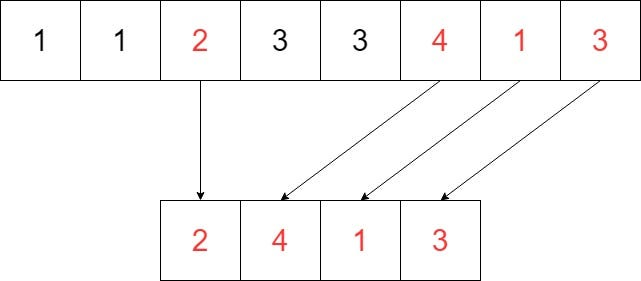Image by Author

As we discussed in our previous article, let’s solve the same problem but using the ArrayList Data Structure.

``````int [] array = {1, 1, 2, 3, 3, 4, 1, 3};
removeDuplicates(array);
``````

This is our original array. And we are calling the method removeDuplicates and passing the original array as a parameter. We then create a method like this.

``````public static void removeDuplicates (int [] arg) { //Solution }
``````

#java-programming #programming-languages #programming #java #arrays #keep the stability of the array